# 置顶贪吃蛇大作战！蛇移动的思考与实现！ Cocos Creator!精选热门

## 【本文使用图文素材转载自微信公众号： 白玉无冰，作者 白玉无冰】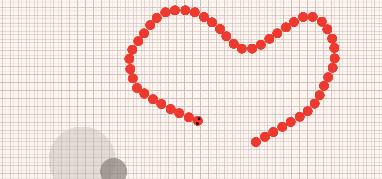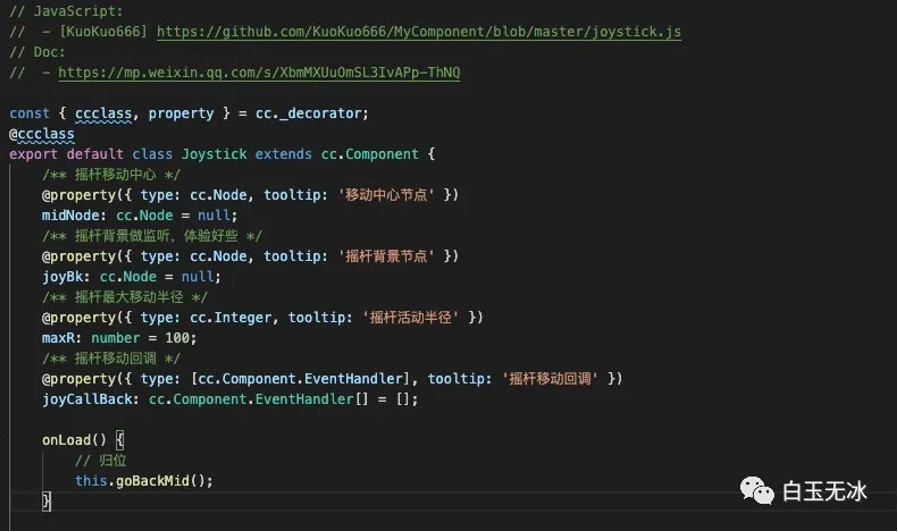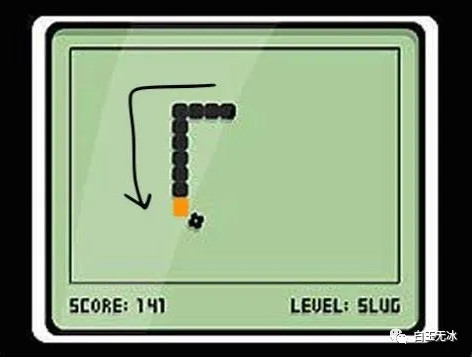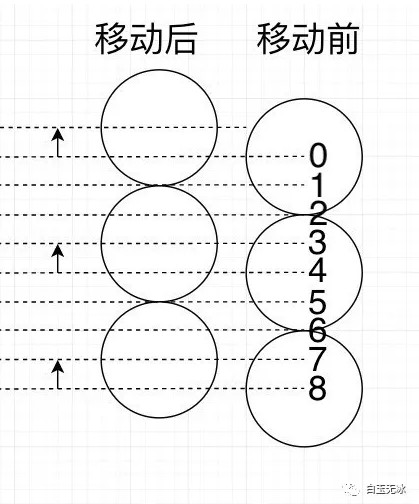```// 蛇总长度
const snake_length = SNAKE_CELL_SIZE * this._node_snake_body.length;
// 每次移动的距离
const snake_move_delta = SNAKE_SPEED * CELL_TIME;
// 总共点数
const snake_pos_count = Math.ceil(snake_length / snake_move_delta) + 1;
this._snake_pos = [];
// 初始化位置信息，按照蛇头的位置往下排
for (let index = 0; index < snake_pos_count; index++) {
}
```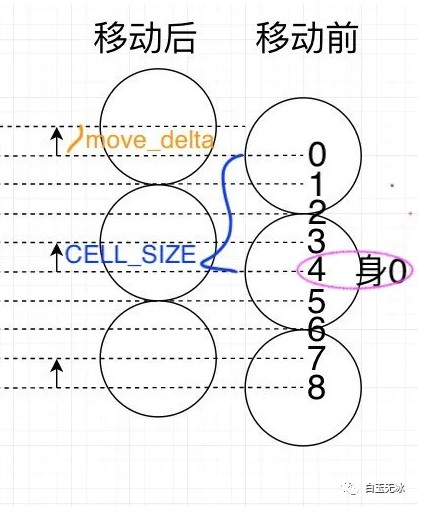```// 每次移动的距离
const snake_move_delta = SNAKE_SPEED * CELL_TIME;
this._node_snake_body.forEach((s, i) => {
// 计算当前身体在位置中的索引
const pos_index = Math.floor((i + 1) * SNAKE_CELL_SIZE / snake_move_delta);
const pos = this._snake_pos[pos_index];
s.x = pos.x;
s.y = pos.y;
})
```

```this.node_snake_head.x += this._snake_vector.x * SNAKE_SPEED * dt;
this.node_snake_head.y += this._snake_vector.y * SNAKE_SPEED * dt;